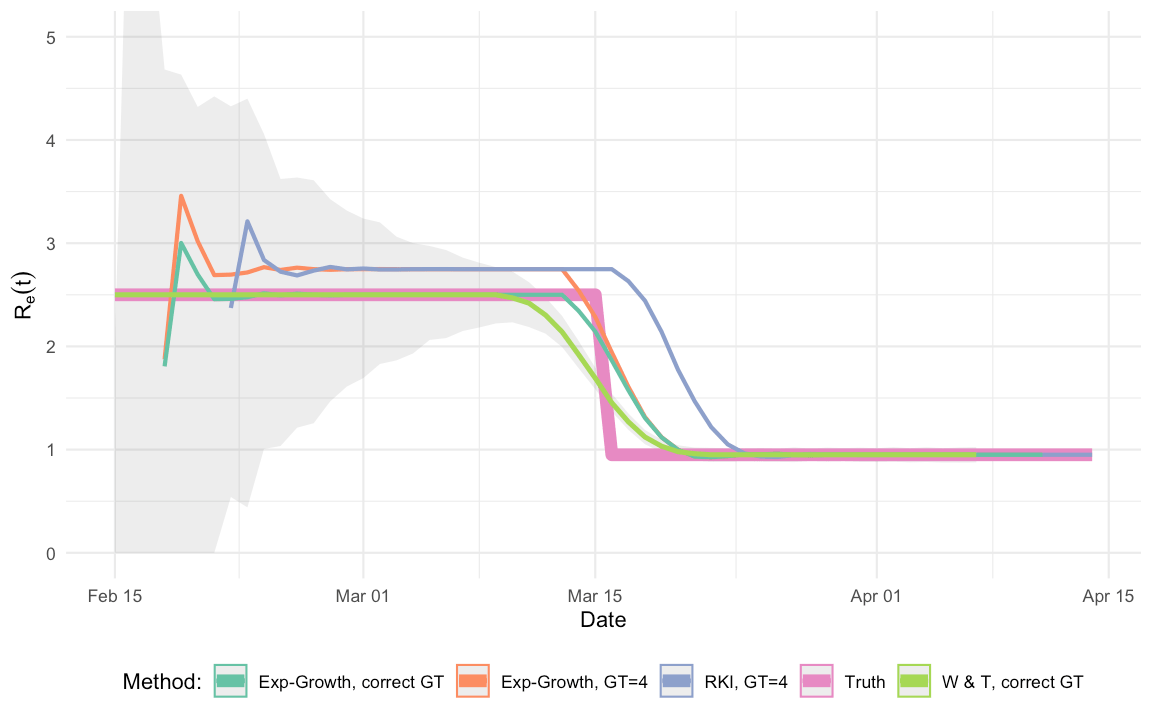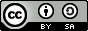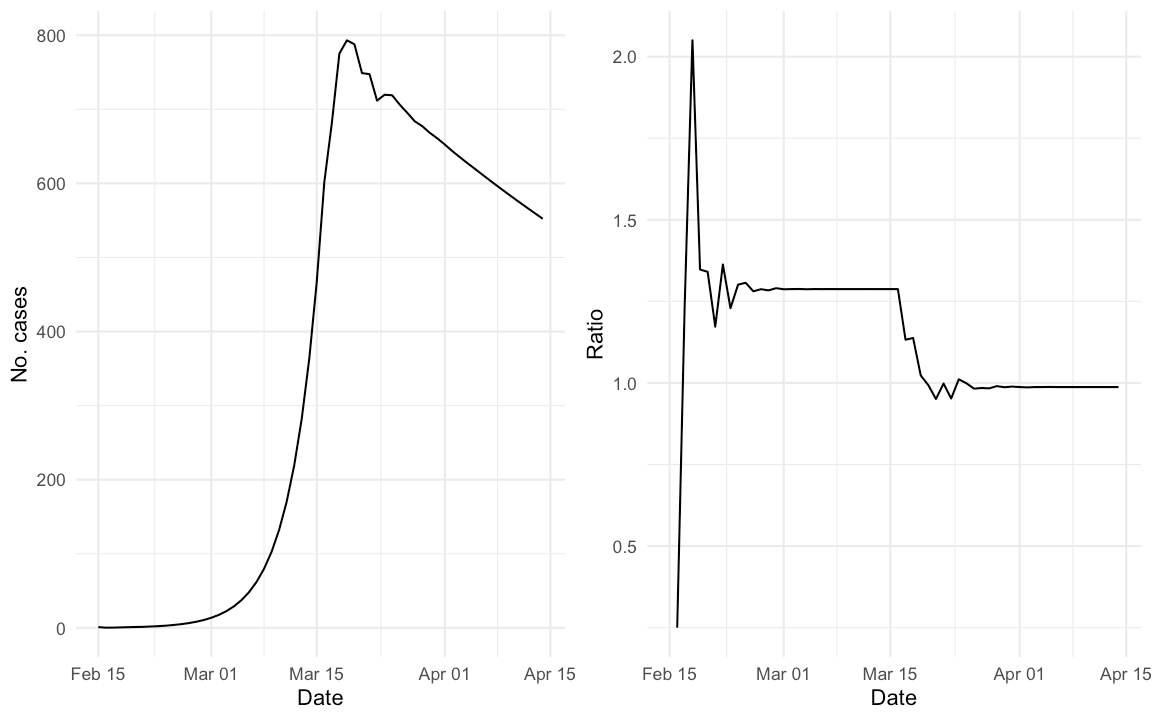Want to share your content on R-bloggers? click here if you have a blog, or here if you don't.

## Abstract:

We discuss the estimation with R of the time-varying effective reproduction number during an infectious disease outbreak such as the COVID-19 outbreak. Using a single simulated outbreak we compare the performance of three different estimation methods.This work is licensed under a Creative Commons Attribution-ShareAlike 4.0 International License. The markdown+Rknitr source code of this blog is available under a GNU General Public License (GPL v3) license from github.

## Motivation

A key parameter to know for an infectious disease pathogen like SARS-nCoV-2 is the basic reproduction number, i.e. the expected number of secondary cases per primary case in a completely susceptible population. This quantity and its relation to the susceptible-infectious-recovered (SIR) model was explained in the previous Flatten the COVID-19 curve post. However, as intervention measures are put in place and as a certain proportion of the population gains immunity, interest switches to knowing the time-varying effective reproduction number. This quantity is defined as follows: Consider an individual, who turns infectious on day $$t$$. We denote by $$R_e(t)$$ the expected number of secondary cases this infectious individual causes. For simplicity we will assume that the timing of being able to infect others and the time of being able to detect this infectivity (e.g. by symptoms or by a test) coincides, i.e. on day $$t$$ the above individual will also appear in the incidence time series. The time between symptom onset in the primary case and symptom onset in the secondary case is called the serial interval. The distribution from which the observed serial intervals origin is called the serial interval distribution. Note that this is different from the generation time, which is the time period between exposure of the primary case and exposure of the secondary case. However, since time of exposure is rarely observable, one instead has to use the time series of incident symptom onsets as basis for inference – see also Svensson (2007) for a thorough discussion of the distinction between the generation time and the serial interval. For the sake of simplicity, but slightly against the reality of SARS-nCoV-2, the remainder of this post will not distinguish between the generation time and the serial interval and will also assume that the serial interval is always positive.

The motivation of this blog post is now to show how to estimate the time-varying effective reproduction number $$R_e(t)$$ using R – in particular the R package R0 (Obadia, Haneef, and Boëlle 2012). The R code of this post is available from github. We shall consider three estimators from the literature. One of the estimators is used by the German Robert-Koch Institute (RKI). Since 8th of April 2020 the estimate is reported as part of their daily COVID-19 situational reports and is thus discussed by major German news media (e.g. ARD).

## Outbreak simulation

We consider a simple growth model and denote by $$y_t$$ the expected number of new symptom onsets we observe on day $$t$$. Let $$(g_1, \ldots, g_M)'$$, denote the probability mass function of the serial interval distribution, i.e. $$P(GT=i) = g_i$$ for $$i=1,2,\ldots, M$$ . The development in the expected number of cases can be described by the homogeneous linear difference equation \begin{align*} y_t = R_e(t-1) g_1 y_{t-1} + \ldots + R_e(t-M) g_M y_{t-M} = \sum_{i=1}^M R_e(t-i) g_i y_{t-i}, \end{align*} where $$t=2, 3, \ldots$$ and where we on the RHS ignore terms when $$t-M \leq 0$$. Furthermore, we fix $$y_1=1$$ and conceptually denote by $$t=1$$ the 15th of February 2020 in calendar time. To simulate a COVID-19 like outbreak with lockdown type intervention we use \begin{align*} R_e(t) = \left\{ \begin{array}{} 2.5 & \text{if } t \leq \text{2020-03-15} \\ 0.95 & \text{otherwise} \end{array} \right. \end{align*}

### Serial Interval Distribution

In what follows we shall use a simple discrete serial interval distribution with support on the values 1-7 days. Such a distribution can easily be stated as

GT_pmf <- structure( c(0, 0.1, 0.1, 0.2, 0.2, 0.2, 0.1, 0.1), names=0:7)
GT_obj <- R0::generation.time("empirical", val=GT_pmf)
GT_obj
## Discretized Generation Time distribution
## mean: 4 , sd: 1.732051
##   0   1   2   3   4   5   6   7
## 0.0 0.1 0.1 0.2 0.2 0.2 0.1 0.1

Note that the selected PMF of the serial interval has mean 4.00 and is symmetric around its mean.

### Outbreak simulation

# Define time varying effective reproduction number
Ret <- function(date) ifelse(date <= as.Date("2020-03-15"), 2.5, 0.95)

# Generate an outbreak (no stochasticity, just the difference equation)
out <- routbreak(n=60, Ret=Ret, GT_obj=GT_obj)
out <- out %>% mutate(ratio = y/lag(y))

We simulate an outbreak using the above difference equation for the selected serial interval distribution starting with one initial case on 2020-02-15. The daily incidence curve of the simulated outbreak looks as follows:For better visualisation the right hand panel shows a plot of $$q_t = y_{t}/y_{t-1}$$. One interesting question is how $$q_t$$ is related to $$R_e(t)$$.

## Wallinga and Teunis (2004)

The method of Wallinga and Teunis (2004) developed as part of the 2003 SARS outbreak is available as function R0::est.R0.TD. It uses a statistical procedure based on the relative likelihood for two cases $$i$$ and $$j$$ with symptom onset at times $$t_i > t_j$$ to be a possible infector-infectee pair. Further methodological details of the method can be found in the paper.

In code:

est_rt_wt <- function(ts, GT_obj) {
end <- length(ts) - (length(GT_objGT)-1) R0::est.R0.TD(ts, GT=GT_obj, begin=1, end=end, nsim=1000) } ### Wallinga and Lipsitch (2006) Wallinga and Lipsitch (2006) discuss the connection between the generation time distribution and the reproduction number. They derive the important relationship that $$R = 1/M(-r)$$, where $$r$$ is the per-capita growth-rate of the epidemic and $$M(\cdot)$$ is the moment generating function of the generation time distribution. As an example, if the generation time distribution is a point-mass distribution with all mass at the value $$G$$ then $$M(u) = \exp(u G)$$. Two possible estimators for $$R$$ in dependence of the generation time would be: \begin{align*} R &= \exp(r \cdot G) & \text{(generation time is a point mass at G)}\\ R &= \left[\sum_{k=1}^\infty \exp(-r \cdot k) \cdot g_k\right]^{-1} & \text{(discrete generation time with PMF } g_1,g_2,\ldots) \end{align*} A simple way to make the above an effective reproduction number estimate is to use a sliding window of half-size $$w$$ centered around time $$t$$ in order to estimate the growth rate parameter $$r$$. For example using a Poisson GLM model of the kind \begin{align*} y_s &\sim \operatorname{Po}(\lambda_s), \text{ with }\\ \log(\lambda_s) &= a + r s, \end{align*} where $$s=t-w,\ldots, t+w$$. An estimator for $$r$$ is then easily extracted together with a confidence interval using a glm approach. In code: #' Window limited exponential growth rate estimator as in #' Wallinga & Lipsitch (2007). #' #' @param ts Time series of incident counts per time interval #' @param GT_obj PMF of the generation time R0.GT object #' @param half_window_width Integer denoting the half-window width est_rt_exp <- function(ts, GT_obj, half_window_width=3L) { # Loop over all time points where the sliding window fits in res <- sapply((half_window_width+1):(length(ts)-half_window_width), function(t) { # Define the sliding window idx <- (t-half_window_width):(t+half_window_width) data <- data.frame(Date=1:length(ts), y=as.numeric(ts)) # Fit a Poisson GLM m <- glm( y ~ 1 + Date, family=poisson, data= data %>% slice(idx)) # Extract the growth rate r <- as.numeric(coef(m)["Date"]) # Equation 2.9 from Wallinga & Lipsitch R <- R.from.r(r, GT_obj) return(R) }) names(res) <- names(ts)[(half_window_width+1):(length(ts)-half_window_width)] return(res) } ## RKI Method In a recent report (an der Heiden and Hamouda 2020) the RKI described their method for computing $$R_e(t)$$ as part of the COVID-19 outbreak as follows (p. 13): For a constant generation time of 4 days, one obtains $$R$$ as the ratio of new infections in two consecutive time periods each consisting of 4 days. Mathematically, this estimation could be formulated as part of a statistical model: \begin{align*} y_{s+4} \>| \> y_s &\sim \operatorname{Po}(R \cdot y_s), \quad s=1,2,3,4. \end{align*} where $$y_1,\ldots, y_4$$ are considered as fixed. From this we obtain $$\hat{R}_{RKI}=\sum_{s=1}^4 y_{s+4} / \sum_{s=1}^4 y_{s}$$. If we use a GLM to fit the model, we not only get $$\hat{R}_{RKI}$$ but also a confidence interval for $$R$$ out-of-the box. Somewhat arbitrary, we denote by $$R_e(t)$$ the above estimate for $$R$$ when $$s=1$$ corresponds to time $$t$$. In code: #' RKI R_e(t) estimator with generation time GT (default GT=4) #' @param ts - Vector of integer values containing the time series of incident cases #' @param GT - PMF of the generation time is a fixed point mass at the value GT. est_rt_rki <- function(ts, GT=4L) { # Sanity check if (!is.integer(GT) | !(GT>0)) stop("GT has to be postive integer.") # Estimate sapply( (1+GT):(length(ts)-GT), function(t) { sum(ts[t+(0:(GT-1))]) / sum(ts[t-(1:GT)]) }) } Basically, the method appears to be the Wallinga and Lipsitch (2006) approach using a point mass generation time distribution, but with a slightly different timing of the sliding windows. However, since no references were given in the RKI publication, I got curious: what happens for this approach when the generation time distribution is not a point mass at $$G$$? What happens if the mean generation time is not an integer? That these are not purely hypothetical question is underlined by the fact that Nishiura, Linton, and Akhmetzhanov (2020) find a serial interval distribution with mean 4.7 days and standard deviation 2.9 as the most suitable fit to data from 28 COVID-19 infector-infectee pairs. Such a serial interval distribution has the following shape:### The Comparison The aim of this post is thus to compare the performance of the RKI estimator with both the Wallinga and Teunis (2004) and the Wallinga and Lipsitch (2006) estimators for the single outbreak simulated in this post. Results do, however, generalize to other configurations. We compute and plot of the $$R_e(t)$$ estimates: # RKI method rt_rki <- est_rt_rki(outy, GT=4L)

# Exponential growth with discrete Laplace transform of a GT \equiv = 4 distribution
rt_exp2 <- est_rt_exp( out$y %>% setNames(out$Date), GT_obj=R0::generation.time("empirical", c(0,0,0,0,1,0,0,0)), half_window_width=3)

# Exponential growth with discrete Laplace transform of the correct GT distribution
rt_exp <- est_rt_exp( out$y %>% setNames(out$Date), GT_obj=GT_obj, half_window_width=3)

# Wallinga Teunis approach with correct GT distribution
rt_wt <- est_rt_wt( out$y, GT=GT_obj) # Data frame with the true values Ret_true <- data.frame(Date=out$Date) %>% mutate(R_hat=Ret(Date), Method="Truth")The shaded area indicates the pointwise confidence intervals of the Wallinga and Teunis (2004) method. To make the results of the graph more explicit, we take a look at 3 time points in the beginning of the outbreak

##         Date W & T, correct GT RKI, GT=4 Truth Exp-G, correct GT
## 1 2020-03-07               2.5  2.748057   2.5          2.500058
## 2 2020-03-08               2.5  2.748072   2.5          2.500011
## 3 2020-03-09               2.5  2.748074   2.5          2.500006

and 3 time points at the end of the outbreak:

##         Date W & T, correct GT RKI, GT=4 Truth Exp-G, correct GT
## 1 2020-04-04              0.95 0.9502227  0.95         0.9498103
## 2 2020-04-05              0.95 0.9501683  0.95         0.9502002
## 3 2020-04-06              0.95 0.9502230  0.95         0.9501288

One observes that the RKI method has a bias in this situation. To some extent this is not surprising, because both the W & T method as well as the exponential growth model use the correct generation time distribution, whereas the RKI method and the exponential growth with point mass distribution use an incorrect serial interval distribution. In practice “the correct” serial interval distribution would not be available – only an estimate. However, one could reflect estimation uncertainty through additional bootstrapping of the two more advanced methods. A more thorough investigation of the methods would of course also have to investigate the effect of misspecification for the W & T (2004) method or the exponential growth approach. The point is, however, that the RKI method as stated in the report is not able to handle a non-point-mass generation time distribution, which does seem necessary. There might be other reasons for choosing such a simplified distribution, but from a statistical point of view it seems like the estimator could be improved.

## Discussion

In this post we showed how to compute the effective reproduction number in R using both own implementations and the R0 package. We illustrated this with data from a hypothetical outbreak. An important message is that there is a mathematical relationship between the daily growth factor and the reproduction number - this relationship is governed by the generation time distribution.

In the present analysis the RKI method, which basically is identical to the approach of inserting the point mass distribution into the formula of Wallinga and Lipsitch (2006), showed a bias when the generation time had the anticipated mean, but had a standard deviation larger than zero. The bias is more pronounced when $$R_e(t)$$ is further away from 1. However, once the lockdown is gradually lifted, $$R_e(t)$$ is likely to raise again making this potentially a relevant bias. The exponential growth approach using the moment generating function of the (discretized) best estimate of the serial interval distribution, can be realized with any statistics package and is computationally fast. Further algorithmic improvements such as Wallinga and Teunis (2004) are readily available in R.

A nice site, which computes time varying reproduction rates for many countries in the world is Temporal variation in transmission during the COVID-19 outbreak by the LSHTM. It is realized using the EpiEstim package (Cori et al. 2013), which also provides $$R_e(t)$$ estimation functions.

## Literature

an der Heiden, M., and O. Hamouda. 2020. “Schätzung Der Aktuel-Len Entwicklung Der Sars-Cov-2-Epidemie in Deutsch-Land – Nowcasting.” Epid Bull 17: 10–15. https://doi.org/10.25646/669.

Cori, Anne, Neil M. Ferguson, Christophe Fraser, and Simon Cauchemez. 2013. “A New Framework and Software to Estimate Time-Varying Reproduction Numbers During Epidemics.” American Journal of Epidemiology 178 (9): 1505–12. https://doi.org/10.1093/aje/kwt133.

Nishiura, Hiroshi, Natalie M. Linton, and Andrei R. Akhmetzhanov. 2020. “Serial Interval of Novel Coronavirus (COVID-19) Infections.” International Journal of Infectious Diseases 93 (April): 284–86. https://doi.org/10.1016/j.ijid.2020.02.060.

Obadia, Thomas, Romana Haneef, and Pierre-Yves Boëlle. 2012. “The R0 Package: A Toolbox to Estimate Reproduction Numbers for Epidemic Outbreaks.” BMC Medical Informatics and Decision Making 12 (1): 147.

Svensson, Å. 2007. “A note on generation times in epidemic models.” Math Biosci 208 (1): 300–311. https://doi.org/10.1016/j.mbs.2006.10.010.

Wallinga, J., and M. Lipsitch. 2006. “How Generation Intervals Shape the Relationship Between Growth Rates and Reproductive Numbers.” Proc. R. Soc. B. 274: 599–604.

Wallinga, J., and P. Teunis. 2004. “Different epidemic curves for severe acute respiratory syndrome reveal similar impacts of control measures.” American Journal of Epidemiology 160 (6): 509–16.